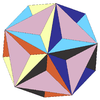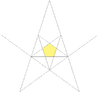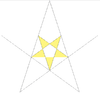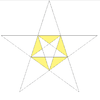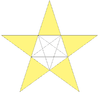# Great dodecahedron

Great dodecahedron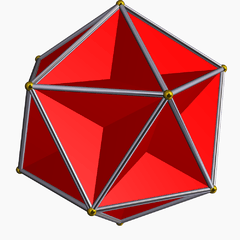TypeKepler–Poinsot polyhedron
Stellation coreregular dodecahedron
ElementsF = 12, E = 30
V = 12 (χ = -6)
Faces by sides12{5}
Schläfli symbol{5,5/2}
Wythoff symbol5/2 | 2 5
Coxeter diagramSymmetry groupIh, H3, [5,3], (*532)
ReferencesU35, C44, W21
PropertiesRegular nonconvex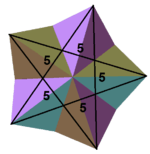(55)/2
(Vertex figure)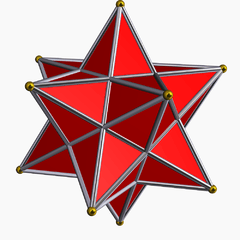Small stellated dodecahedron
(dual polyhedron)

In geometry, the great dodecahedron is a Kepler–Poinsot polyhedron, with Schläfli symbol {5,5/2} and Coxeter–Dynkin diagram of. It is one of four nonconvex regular polyhedra. It is composed of 12 pentagonal faces (six pairs of parallel pentagons), with five pentagons meeting at each vertex, intersecting each other making a pentagrammic path.

The discovery of the great dodecahedron is sometimes credited to Louis Poinsot in 1810, though there is a drawing of something very similar to a great dodecahedron in the 1568 book Perspectiva Corporum Regularium by Wenzel Jamnitzer.

## Images

Transparent model Spherical tiling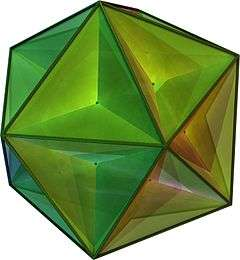(With animation)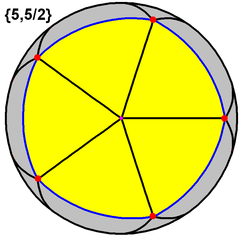This polyhedron represents a spherical tiling with a density of 3. (One spherical pentagon face is shown above in yellow)
Net Stellation× 20
Net for surface geometry; twenty isosceles triangular pyramids, arranged like the faces of an icosahedron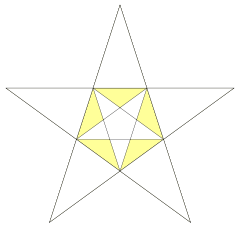It can also be constructed as the second of three stellations of the dodecahedron, and referenced as Wenninger model [W21].

## Related polyhedra

It shares the same edge arrangement as the convex regular icosahedron.

If the great dodecahedron is considered as a properly intersected surface geometry, it has the same topology as a triakis icosahedron with concave pyramids rather than convex ones.

A truncation process applied to the great dodecahedron produces a series of nonconvex uniform polyhedra. Truncating edges down to points produces the dodecadodecahedron as a rectified great dodecahedron. The process completes as a birectification, reducing the original faces down to points, and producing the small stellated dodecahedron.

Name Small stellated dodecahedron Dodecadodecahedron Truncated
great
dodecahedron
Great
dodecahedron
Coxeter-Dynkin
diagramPicture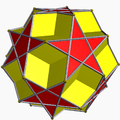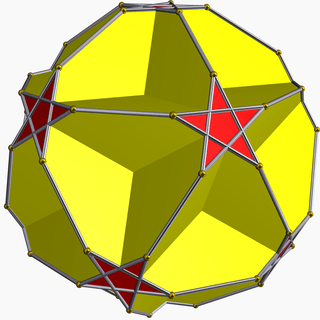## Usage

Dodecahedron Small stellated dodecahedron Great dodecahedron Great stellated dodecahedron Stellations of the dodecahedron Platonic solid Kepler–Poinsot solids